# RRB ALP Recruitment – Important Geometry Formulas

RRB ALP Recruitment and Group D Exam is nearing. It is a golden opportunity to get a Job with Indian Railways. To be able to clear the RRB ALP and Group D Recruitment Exam what is required is a good preparation plan. To assist you with your preparation, In this blog, we have compiled a list of important Geometrical formulas.RRB ALP Recruitment and Group D Exam – Formulas of Area and Perimeter

 Figure Area Perimeter Circle -: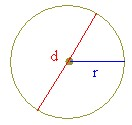= pi × r2 or = (pi × d2) / 4 = 2 × pi × r or = pi × d Square -:= s2 = s + s + s + s = 4 × s Rectangle -: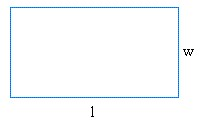= l × w = l + l + w + w = 2 × l + 2 × w Triangle -: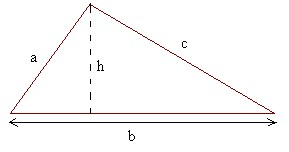= (b × h) / 2 = a + b + c Parallelogram -: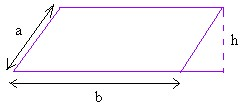= b × h = a + a + b + b = 2 × a + 2 × b Rhombus -: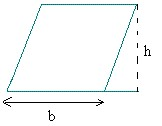= b × h = b + b + b + b = 4 × b Trapezoid -: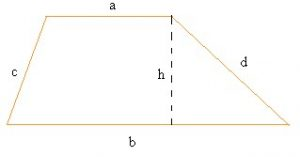= (a + b) / 2 × h = a + b + c + dRRB ALP Recruitment and Group D Exam – Sample Questions

Q1) The width of a rectangular hall is 3/4th of its length. If the area of the hall is 300 sq.m, then the difference between its length and width is:

(a) 3 m

(b) 4 m

(c) 5 m

(d) 15 m

Answer = Option (c) – 5 m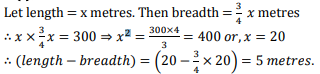Q2) A man walked 20 m to cross a rectangular field diagonally. If the length of the field is 16 m, the breadth of the field is:

(a) 4 m

(b) 16 m

(c) 12 m

(d) Can’t be determined

Answer = Option (c) – 12 m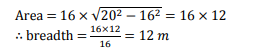We hope this blog is helpful for your RRB ALP Recruitment and Group D Exam preparation.If any have any further queries or suggestions regarding RRB ALP Recruitment and Group D Exam you can connect with us on FBTwitterInstagram and YouTube as well.

All the best for RRB ALP Recruitment and Group D Exam

Related Articles: